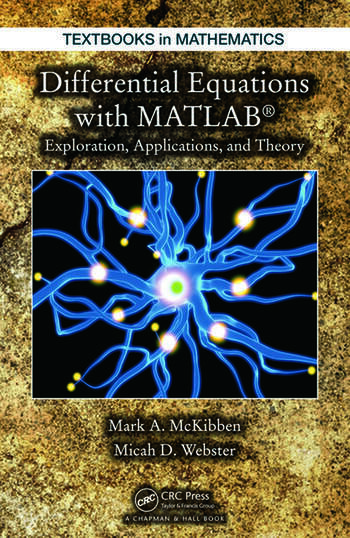# Differential Equations with MATLAB: Exploration, Applications, and Theory

## 1st Edition

Mark McKibben, Micah D. Webster

Chapman and Hall/CRC
Published September 8, 2014
Textbook - 497 Pages - 215 B/W Illustrations
ISBN 9781466557079 - CAT# K15453
Series: Textbooks in Mathematics

For Instructors Request Inspection Copy

USD\$125.00

FREE Standard Shipping!

## Preview

### Summary

A unique textbook for an undergraduate course on mathematical modeling, Differential Equations with MATLAB: Exploration, Applications, and Theory provides students with an understanding of the practical and theoretical aspects of mathematical models involving ordinary and partial differential equations (ODEs and PDEs). The text presents a unifying picture inherent to the study and analysis of more than 20 distinct models spanning disciplines such as physics, engineering, and finance.

The first part of the book presents systems of linear ODEs. The text develops mathematical models from ten disparate fields, including pharmacokinetics, chemistry, classical mechanics, neural networks, physiology, and electrical circuits. Focusing on linear PDEs, the second part covers PDEs that arise in the mathematical modeling of phenomena in ten other areas, including heat conduction, wave propagation, fluid flow through fissured rocks, pattern formation, and financial mathematics.

The authors engage students by posing questions of all types throughout, including verifying details, proving conjectures of actual results, analyzing broad strokes that occur within the development of the theory, and applying the theory to specific models. The authors’ accessible style encourages students to actively work through the material and answer these questions. In addition, the extensive use of MATLAB® GUIs allows students to discover patterns and make conjectures.

#### Instructors

We provide complimentary e-inspection copies of primary textbooks to instructors considering our books for course adoption.